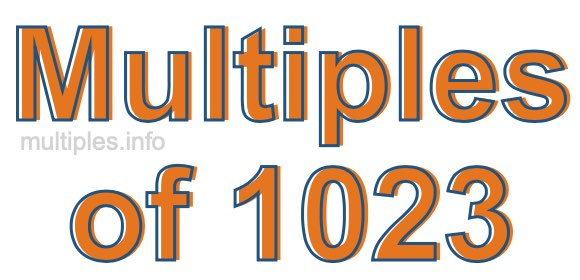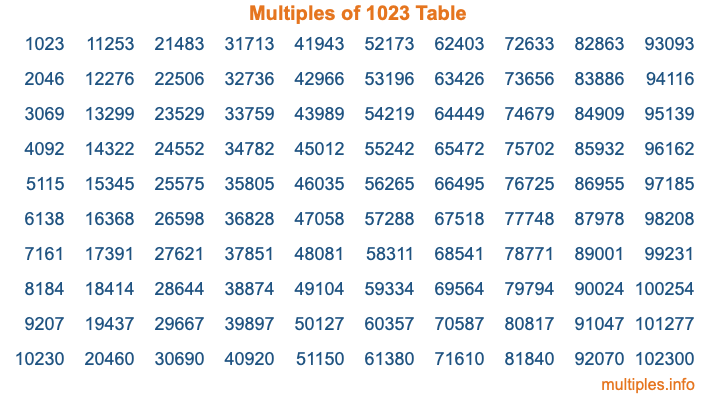Multiples of 1023Welcome to the Multiples of 1023 page. Here we will first teach you everything you will ever need to know about the multiples of 1023, and then give you a study guide summary of everything we taught you to make sure you remember it all. Use this page to look up facts and learn information about the multiples of 1023. This page will make you a multiples of one thousand twenty-three expert!

Definition of Multiples of 1023
Multiples of 1023 are all the numbers that when divided by 1023 equal an integer. Each of the multiples of 1023 are called a multiple. A multiple of 1023 is created by multiplying 1023 by an integer.

Therefore, to create a list of multiples of 1023, you start with 1 multiplied by 1023, then 2 multiplied by 1023, then 3 multiplied by 1023, and so on for as long as you want. Thus, the list of the first five multiples of 1023 is 1023, 2046, 3069, 4092, and 5115. To see a larger list of multiples of 1023, see the printable image of Multiples of 1023 further down on this page. We also have a category where you can choose any nth multiple of 1023.

Multiples of 1023 Checker
The Multiples of 1023 Checker below checks to see if any number of your choice is a multiple of 1023. In other words, it checks to see if there is any number (integer) that when multiplied by 1023 will equal your number. To do that, we divide your number by 1023. If the the quotient is an integer, then your number is a multiple of 1023.

Is  a multiple of 1023?

Least Common Multiple of 1023 and ...
A Least Common Multiple (LCM) is the lowest multiple that two or more numbers have in common. This is also called the smallest common multiple or lowest common multiple and is useful to know when you are adding our subtracting fractions. Enter one or more numbers below (1023 is already entered) to find the LCM.

Check out our LCM Calculator if you need more details about the Least Common Multiple or if you need the LCM for different numbers for adding and subtraction fractions.

nth Multiple of 1023
As we stated above, 1023 is the first multiple of 1023, 2046 is the second multiple of 1023, 3069 is the third multiple of 1023, and so on. Enter a number below to find the nth multiple of 1023.

th multiple of 1023

Multiples of 1023 vs Factors of 1023
1023 is a multiple of 1023 and a factor of 1023, but that is where the similarities end. All postive multiples of 1023 are 1023 or greater than 1023. All positive factors of 1023 are 1023 or less than 1023.

Below is the beginning list of multiples of 1023 and the factors of 1023 so you can compare:

Multiples of 1023: 1023, 2046, 3069, 4092, 5115, etc.

Factors of 1023: 1, 3, 11, 31, 33, 93, 341, 1023

As you can see, the multiples of 1023 are all the numbers that you can divide by 1023 to get a whole number. The factors of 1023, on the other hand, are all the whole numbers that you can multiply by another whole number to get 1023.

It's also interesting to note that if a number (x) is a factor of 1023, then 1023 will also be a multiple of that number (x).

Multiples of 1023 vs Divisors of 1023
The divisors of 1023 are all the integers that 1023 can be divided by evenly. Below is a list of the divisors of 1023.

Divisors of 1023: 1, 3, 11, 31, 33, 93, 341, 1023

The interesting thing to note here is that if you take any multiple of 1023 and divide it by a divisor of 1023, you will see that the quotient is an integer.

Multiples of 1023 Table
Below is an image of the first 100 multiples of 1023 in a table. The table is in chronological order, column by column. The first column has the first ten multiples of 1023, the second column has the next ten multiples of 1023, and so on.The Multiples of 1023 Table is also referred to as the 1023 Times Table or Times Table of 1023. You are welcome to print out our table for your studies.

Negative Multiples of 1023
Although not often discussed or needed in math, it is worth mentioning that you can make a list of negative multiples of 1023 by multiplying 1023 by -1, then by -2, then by -3, and so on, to get the following list of negative multiples of 1023:

-1023, -2046, -3069, -4092, -5115, etc.

Multiples of 1023 Summary
Below is a summary of important Multiples of 1023 facts that we have discussed on this page. To retain the knowledge on this page, we recommend that you read through the summary and explain to yourself or a study partner why they hold true.

There are an infinite number of multiples of 1023.

A multiple of 1023 divided by 1023 will equal a whole number.

1023 divided by a factor of 1023 equals a divisor of 1023.

The nth multiple of 1023 is n times 1023.

The largest factor of 1023 is equal to the first positive multiple of 1023.

1023 is a multiple of every factor of 1023.

1023 is a multiple of 1023.

A multiple of 1023 divided by a divisor of 1023 equals an integer.

1023 divided by a divisor of 1023 equals a factor of 1023.

Any integer times 1023 will equal a multiple of 1023.

Multiples of a Number
Here you can get the multiples of another number, all with the same attention to detail as we did for multiples of 1023 on this page.

Multiples of
Multiples of 1024
Did you find our page about multiples of one thousand twenty-three educational? Do you want more knowledge? Check out the multiples of the next number on our list!

Copyright  |   Privacy Policy  |   Disclaimer  |   Contact